### IMO Shortlist 2017 problem N3

Kvaliteta:
Avg: 0,0
Težina:
Avg: 7,0

Determine all integers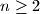$n\geq 2$ having the following property: for any integers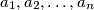$a_1,a_2,\ldots, a_n$ whose sum is not divisible by$n$, there exists an index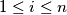$1 \leq i \leq n$ such that none of the numbers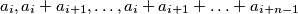is divisible by$n$. Here, we let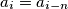$a_i=a_{i-n}$ when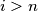$i >n$.

Izvor: https://www.imo-official.org/problems/IMO2017SL.pdf